# ISEE Upper Level Quantitative : How to find the common part of a Venn Diagram

## Example Questions

### Example Question #1 : How To Find The Common Part Of A Venn Diagram

What is the greatest common factor ofand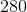?Explanation:

To solve for the greatest common factor, it is necessary to get your numbers into prime factor form. For each of your numbers, this is: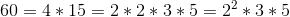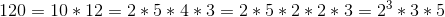Next, for each of your sets of prime factors, you need to choose the exponent for which you have the smallest value; therefore, for your values, you choose: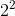Taking these together, you get: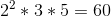### Example Question #2 : How To Find The Common Part Of A Venn Diagram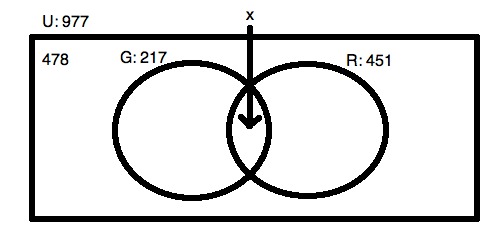For the Venn Diagram pictured above, what is the valuefor the overlap of the two sets drawn as circles?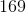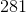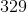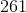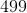Explanation:

Solving for the overlap of two sets is easy when you have all of your data. You know that the two circles added up will have to equal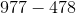or. This is the total amount in the "universe" (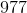minus the amount that is found outside of the two circles (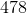).

Because the overlap happens once in each circle, you know that: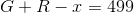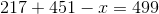Solving, this means: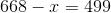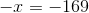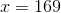### Example Question #1 : How To Find The Common Part Of A Venn Diagram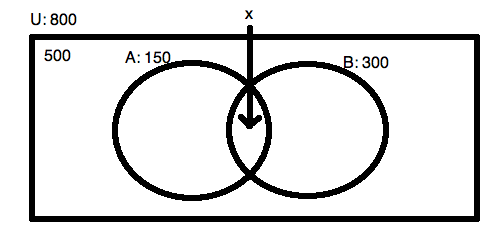For the Venn Diagram pictured above, what is the valuefor the overlap of the two sets drawn as circles?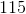Explanation:

Solving for the overlap of two sets is easy when you have all of your data. You know that the two circles added up will have to equal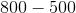or. This is the total amount in the "universe" (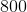minus the amount that is found outside of the two circles (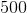).

Because the overlap happens once in each circle, you know that: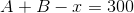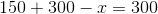Solving, this means:. The overlap is the whole of circle!

### Example Question #1 : How To Find The Common Part Of A Venn Diagram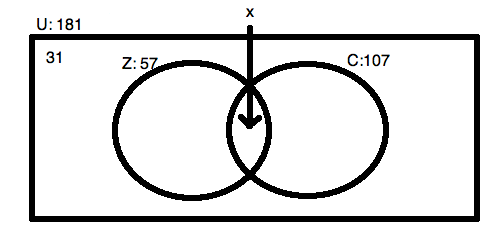For the Venn Diagram pictured above, what is the valuefor the overlap of the two sets drawn as circles?Explanation:

Solving for the overlap of two sets is easy when you have all of your data. You know that the two circles added up will have to equal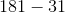or.  This is the total amount in the "universe" (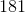minus the amount that is found outside of the two circles ().

Because the overlap happens once in each circle, you know that: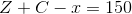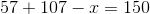Solving, this means: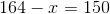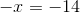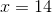### Example Question #5 : How To Find The Common Part Of A Venn Diagram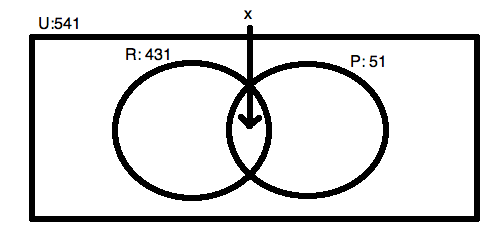For the Venn Diagram pictured above, what is the valuefor the overlap of the two sets drawn as circles?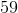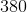Do not be tricked by this question! In order to solve for the overlap, you need to know the amount that is in the area outside of the circles but still inside the universal box area! You cannot figure out the answer without knowing this fact; therefore, you must select "No answer is possible."  We know that the two circles do not exhaust the universe because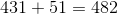. This is not large enough to fill the complete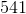. If it were, you would know that the overlap is.